Publicité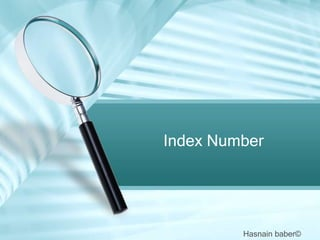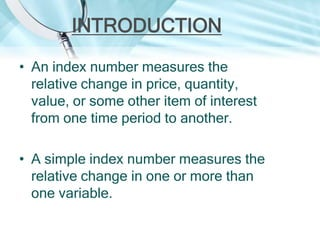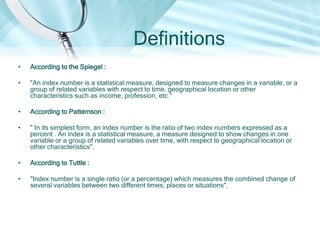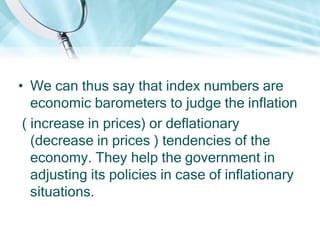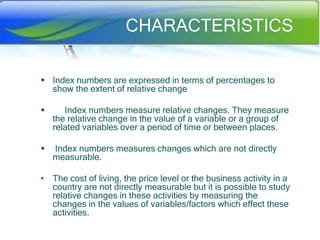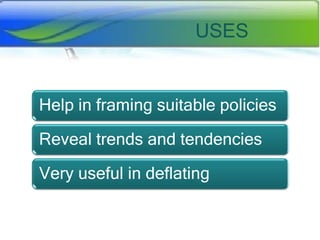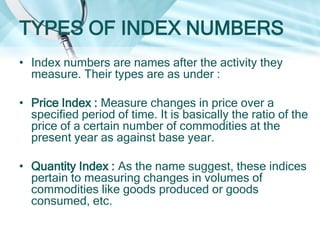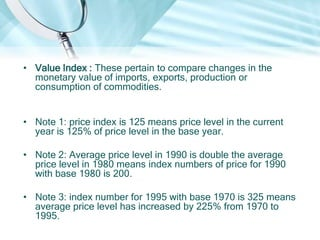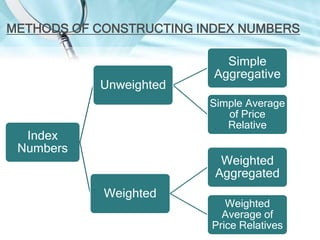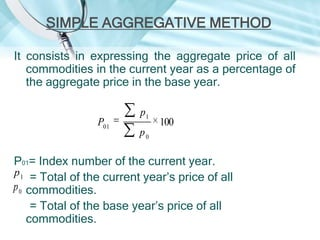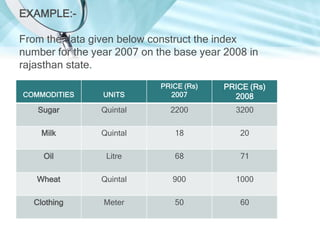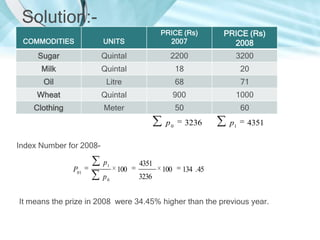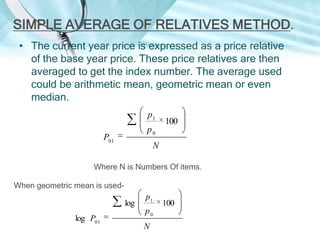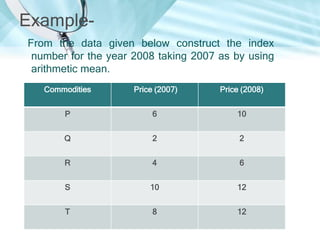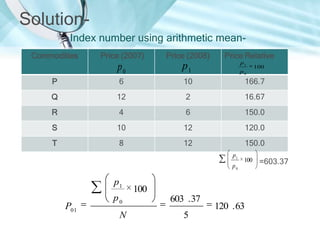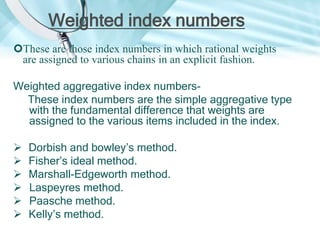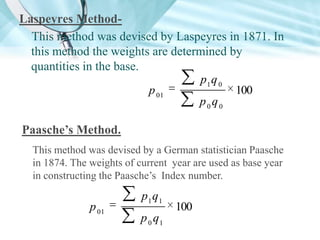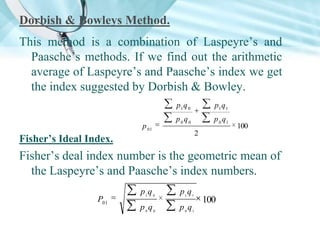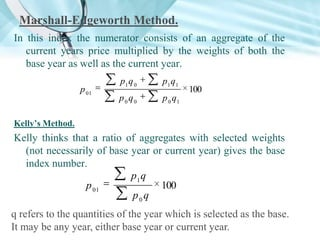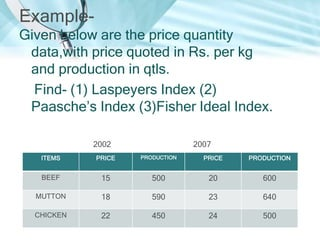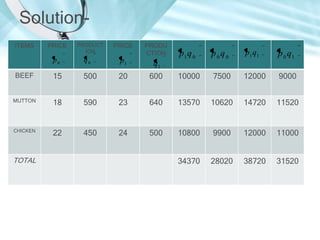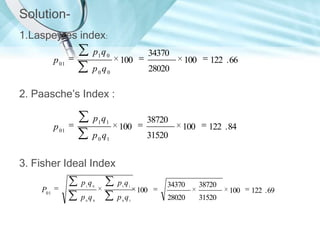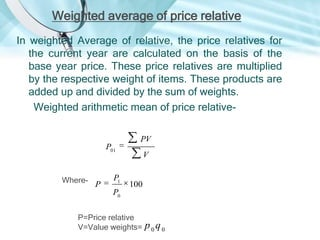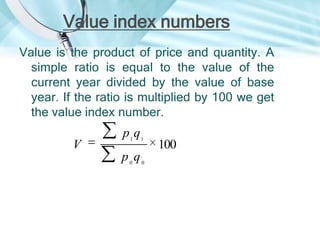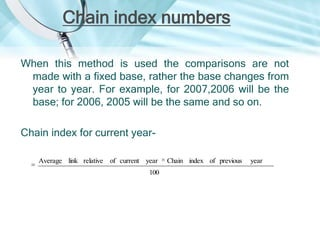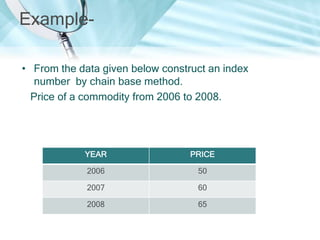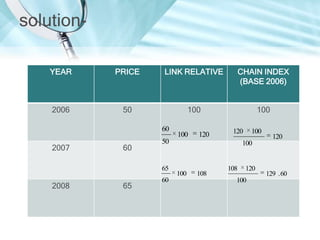1 sur 27
Publicité

### Index number

2. INTRODUCTION • An index number measures the relative change in price, quantity, value, or some other item of interest from one time period to another. • A simple index number measures the relative change in one or more than one variable.
3. Definitions • According to the Spiegel : • "An index number is a statistical measure, designed to measure changes in a variable, or a group of related variables with respect to time, geographical location or other characteristics such as income, profession, etc.“ • According to Patternson : • " In its simplest form, an index number is the ratio of two index numbers expressed as a percent . An index is a statistical measure, a measure designed to show changes in one variable or a group of related variables over time, with respect to geographical location or other characteristics". • According to Tuttle : • "Index number is a single ratio (or a percentage) which measures the combined change of several variables between two different times, places or situations".
4. • We can thus say that index numbers are economic barometers to judge the inflation ( increase in prices) or deflationary (decrease in prices ) tendencies of the economy. They help the government in adjusting its policies in case of inflationary situations.
5. CHARACTERISTICS  Index numbers are expressed in terms of percentages to show the extent of relative change  Index numbers measure relative changes. They measure the relative change in the value of a variable or a group of related variables over a period of time or between places.  Index numbers measures changes which are not directly measurable. • The cost of living, the price level or the business activity in a country are not directly measurable but it is possible to study relative changes in these activities by measuring the changes in the values of variables/factors which effect these activities.
6. USES Help in framing suitable policies Reveal trends and tendencies Very useful in deflating
7. TYPES OF INDEX NUMBERS • Index numbers are names after the activity they measure. Their types are as under : • Price Index : Measure changes in price over a specified period of time. It is basically the ratio of the price of a certain number of commodities at the present year as against base year. • Quantity Index : As the name suggest, these indices pertain to measuring changes in volumes of commodities like goods produced or goods consumed, etc.
8. • Value Index : These pertain to compare changes in the monetary value of imports, exports, production or consumption of commodities. • Note 1: price index is 125 means price level in the current year is 125% of price level in the base year. • Note 2: Average price level in 1990 is double the average price level in 1980 means index numbers of price for 1990 with base 1980 is 200. • Note 3: index number for 1995 with base 1970 is 325 means average price level has increased by 225% from 1970 to 1995.
9. METHODS OF CONSTRUCTING INDEX NUMBERS Unweighted Simple Aggregative Simple Average of Price Relative Index Numbers Weighted Aggregated Weighted Weighted Average of Price Relatives
10. SIMPLE AGGREGATIVE METHOD It consists in expressing the aggregate price of all commodities in the current year as a percentage of the aggregate price in the base year. P01 p1 100 p0 P01= Index number of the current year. p 1 = Total of the current year’s price of all p 0 commodities. = Total of the base year’s price of all commodities.
11. EXAMPLE:From the data given below construct the index number for the year 2007 on the base year 2008 in rajasthan state. COMMODITIES UNITS PRICE (Rs) 2007 Sugar Quintal 2200 3200 Milk Quintal 18 20 Oil Litre 68 71 Wheat Quintal 900 1000 Clothing Meter 50 60 PRICE (Rs) 2008
12. Solution:COMMODITIES UNITS PRICE (Rs) 2007 Sugar Quintal 2200 3200 Milk Quintal 18 20 Oil Litre 68 71 Wheat Quintal 900 1000 Clothing Meter 50 60 p0 3236 100 PRICE (Rs) 2008 134 . 45 p1 4351 Index Number for 2008P01 p1 p0 100 4351 3236 It means the prize in 2008 were 34.45% higher than the previous year.
13. SIMPLE AVERAGE OF RELATIVES METHOD. • The current year price is expressed as a price relative of the base year price. These price relatives are then averaged to get the index number. The average used could be arithmetic mean, geometric mean or even median. p1 100 p0 P01 N Where N is Numbers Of items. When geometric mean is used- log log P01 p1 p0 N 100
14. ExampleFrom the data given below construct the index number for the year 2008 taking 2007 as by using arithmetic mean. Commodities Price (2007) Price (2008) P 6 10 Q 2 2 R 4 6 S 10 12 T 8 12
15. SolutionIndex number using arithmetic meanCommodities Price (2007) Price (2008) Price Relative p0 p1 P 6 10 166.7 Q 12 2 16.67 R 4 6 150.0 S 10 12 120.0 T 8 12 150.0 p1 p1 100 p0 p1 P01 p0 N 100 603 . 37 5 100 p0 120 . 63 =603.37
16. Weighted index numbers These are those index numbers in which rational weights are assigned to various chains in an explicit fashion. Weighted aggregative index numbersThese index numbers are the simple aggregative type with the fundamental difference that weights are assigned to the various items included in the index.       Dorbish and bowley’s method. Fisher’s ideal method. Marshall-Edgeworth method. Laspeyres method. Paasche method. Kelly’s method.
17. Laspeyres MethodThis method was devised by Laspeyres in 1871. In this method the weights are determined by quantities in the base. p1 q 0 p 01 100 p0q0 Paasche’s Method. This method was devised by a German statistician Paasche in 1874. The weights of current year are used as base year in constructing the Paasche’s Index number. p 01 p1 q1 p 0 q1 100
18. Dorbish & Bowleys Method. This method is a combination of Laspeyre’s and Paasche’s methods. If we find out the arithmetic average of Laspeyre’s and Paasche’s index we get the index suggested by Dorbish & Bowley. p1 q 0 p 01 p1 q1 p0q0 p 0 q1 100 2 Fisher’s Ideal Index. Fisher’s deal index number is the geometric mean of the Laspeyre’s and Paasche’s index numbers. P01 p1q 0 p1 q1 p0q0 p 0 q1 100
19. Marshall-Edgeworth Method. In this index the numerator consists of an aggregate of the current years price multiplied by the weights of both the base year as well as the current year. p 01 p1 q 0 p1 q1 p0q0 p 0 q1 100 Kelly’s Method. Kelly thinks that a ratio of aggregates with selected weights (not necessarily of base year or current year) gives the base index number. p1 q p 01 100 p0q q refers to the quantities of the year which is selected as the base. It may be any year, either base year or current year.
20. ExampleGiven below are the price quantity data,with price quoted in Rs. per kg and production in qtls. Find- (1) Laspeyers Index (2) Paasche’s Index (3)Fisher Ideal Index. 2002 2007 ITEMS PRICE PRODUCTION PRICE PRODUCTION BEEF 15 500 20 600 MUTTON 18 590 23 640 CHICKEN 22 450 24 500
21. SolutionITEMS PRICE PRODUCT ION PRICE PRODU CTION p1 q 0 p0q0 p1 q1 p 0 q1 p0 q0 p1 q1 BEEF 15 500 20 600 10000 7500 12000 9000 MUTTON 18 590 23 640 13570 10620 14720 11520 CHICKEN 22 450 24 500 10800 9900 12000 11000 34370 28020 38720 31520 TOTAL
22. Solution1.Laspeyres index: p 01 p1 q 0 34370 100 p0q0 100 122 . 66 100 122 . 84 28020 2. Paasche’s Index : p 01 p1 q1 100 p 0 q1 38720 31520 3. Fisher Ideal Index P01 p1q 0 p1 q1 p0q0 p 0 q1 100 34370 38720 28020 31520 100 122 . 69
23. Weighted average of price relative In weighted Average of relative, the price relatives for the current year are calculated on the basis of the base year price. These price relatives are multiplied by the respective weight of items. These products are added up and divided by the sum of weights. Weighted arithmetic mean of price relative- P01 Where- P P1 PV V 100 P0 P=Price relative V=Value weights= p0q0
24. Value index numbers Value is the product of price and quantity. A simple ratio is equal to the value of the current year divided by the value of base year. If the ratio is multiplied by 100 we get the value index number. V p1 q1 p0q0 100
25. Chain index numbers When this method is used the comparisons are not made with a fixed base, rather the base changes from year to year. For example, for 2007,2006 will be the base; for 2006, 2005 will be the same and so on. Chain index for current yearAverage link relative of current year 100 Chain index of previous year
26. Example• From the data given below construct an index number by chain base method. Price of a commodity from 2006 to 2008. YEAR PRICE 2006 50 2007 60 2008 65
27. solutionYEAR PRICE LINK RELATIVE CHAIN INDEX (BASE 2006) 2006 50 100 100 60 2007 60 65 120 120 50 65 2008 100 60 100 120 100 100 108 108 120 100 129 . 60
Publicité